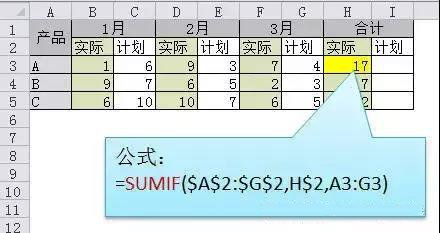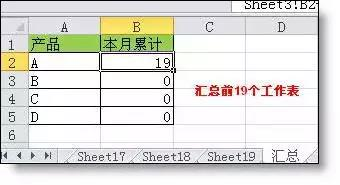#### Excel多表多位置计算公式

1、隔列求和公式

=SUMIF(\$A\$2:\$G\$2,H\$2,A3:G3)

=SUMPRODUCT((MOD(COLUMN(B3:G3),2)=0)*B3:G3)2、多表相同位置求和

=SUM(Sheet1:Sheet19!B2)3、两条查找相同公式

=COUNTIF(Sheet15!A:A,A2)4、两表数据多条件核对

=SUMPRODUCT((\$A\$2:\$A\$6=A10)*(\$B\$2:\$B\$6=B10)*\$C\$2:\$C\$6)-C105、个税计算

=5*MAX(A2*{0.6,2,4,5,6,7,9}%-{21,91,251,376,761,1346,3016},)